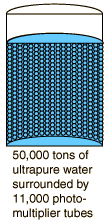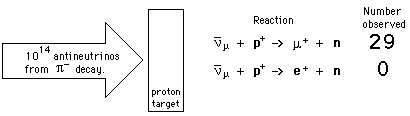# Super-Kamiokande Neutrino Detector

Inside Mount Ikenoyama in Japan within an active zinc mine is a remarkable tank of ultrapure water which is the world's most sensitive neutrino detector as of 1999.The 50,000 tons of water in the tank detector is so pure and transparent that light passes 70 meters before its intensity drops to half, compared to a few meters in a typical swimming pool. The 11,000 hand-blown photomultiplier tubes are half a meter in diameter and coated inside with a thin layer of alkali metal to detect the Cerenkov radiation from the interactions of either electron neutrinos or muon neutrinos. Kearns, Kajita and Totsuka report the detection of 5000 neutrino events in two years of measurement. From the nature of the interactions of cosmic rays in the upper atmosphere, two muon neutrinos are expected for every electron neutrino, but they found a ratio of only 1.3 to 1. Their interpretation of this result is that the deficiency is caused by "neutrino oscillation" in which a number of muon neutrinos are transmuted into tau neutrinos which are undetectable. Neutrino oscillation has been hotly debated over the past few years because its existence implies a mass for the neutrinos. Further detailed analysis of this data from Super-Kamiokande is consistent with neutrino oscillation, so this is the first clear experimental evidence supporting a non-zero mass for the neutrino.
 Does the neutrino have any mass?
 Accident at Super-Kamiokande
Index

Particle concepts

Reference
Kearns, et al.

 HyperPhysics***** Quantum Physics R Nave
Go Back

# Electron, Muon, and Tau Neutrinos

The massive leptons are the electron, muon and tau, and each of them has an associated neutrino. Most experiments involving neutrinos involve electron neutrinos which are much more common in our low-energy world, but some current neutrino detectors are sensitive to the other two as well. The current generation of neutrino detectors such as the Super-Kamiokande can detect and distinguish between electron and muon neutrinos. When a muon neutrino interacts with a nucleus, it can produce an energetic muon which travels only a short distance, emitting a sharply outlined cone of Cerenkov radiation which can be detected by photomultiplier tubes. An electron neutrino interaction can produce an energetic electron, but the Cerenkov cone from this interaction differs significantly from that of the muon. The electron generates a shower of electrons and positrons, each with its own Cerenkov cone. This smears out the circle of light which hits the detectors; the diffuse circle at the photomultipliers is the signature of the electron neutrino. Tau neutrinos are not detected by these detectors because the neutrino energies are not sufficient to produce tau particles (which are about 3500 times the mass of an electron).

The first clear experimental evidence for the difference between electron and muon neutrinos came from an experiment conducted at Brookhaven in 1962. The two reactions indicated would have been equally probable if electron and muon neutrinos were the same.G. Danby, et al.,Phys. Rev. Lett. 9,36 (1962) . Also see L. Lederman, Scientific American, (March 1963).

Since the electron and muon neutrinos are distinct, the second reaction above violates conservation of lepton number.

Index

Particle concepts

Reference
Kearns, et al.

Griffiths
Sec 1.5

 HyperPhysics***** Quantum Physics R Nave
Go Back